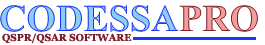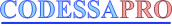Constitutional Topological Geometrical Electrostatic CPSA Quantum-chemical MO-related Thermodynamic

Quantum Chemical Descriptors

Total energy of the molecule

Total electronic energy of the molecule

Standard heat of formation

Electron-electron repulsion energy for a given atomic species

Nuclear-electron attraction energy for a given atomic species

Electron-electron repulsion between two given atoms

Nuclear-electron attraction energy between two given atoms

Nuclear repulsion energy between two given atoms

Total electrostatic interaction energy between two given atomic species

Total interaction energy between two given two atomic species

Total molecular one-center electron-electron repulsion energy

Total molecular one-center electron-nuclear attraction energy

Total intramolecular electrostatic interaction energy

Electron kinetic energy density

Energy of protonationUniversity of Florida 2001. All rights reserved